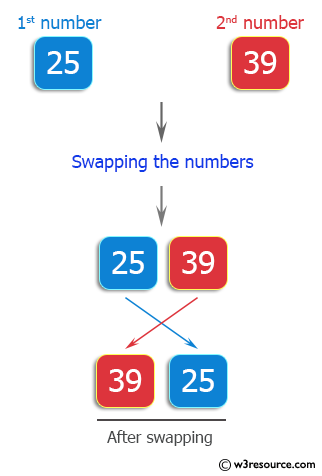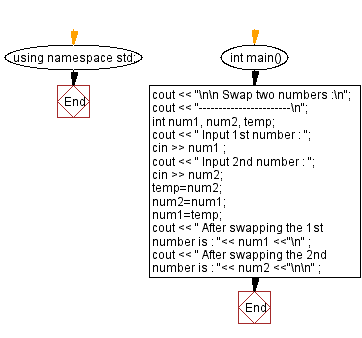﻿ C++ Exercises: Swap two numbers - w3resource

# C++ Exercises: Swap two numbers

## C++ Basic: Exercise-13 with Solution

Write a C++ program that swaps two numbers.

Pictorial Presentation:Sample Solution :-

C++ Code :

``````#include <iostream>
using namespace std;

int main()
{
cout << "\n\n Swap two numbers :\n";
cout << "-----------------------\n";
int num1, num2, temp;
cout << " Input 1st number : ";
cin >> num1 ;
cout << " Input 2nd number : ";
cin >> num2;
temp=num2;
num2=num1;
num1=temp;
cout << " After swapping the 1st number is : "<< num1 <<"\n" ;
cout << " After swapping the 2nd number is : "<< num2 <<"\n\n" ;
}
``````

Sample Output:

``` Swap two numbers :
-----------------------
Input 1st number : 25
Input 2nd number : 39
After swapping the 1st number is : 39
After swapping the 2nd number is : 25
```

Flowchart:C++ Code Editor:

What is the difficulty level of this exercise?

﻿

## C++ Programming: Tips of the Day

Why is there no std::stou?

The most pat answer would be that the C library has no corresponding "strtou", and the C++11 string functions are all just thinly veiled wrappers around the C library functions: The std::sto* functions mirror strto*, and the std::to_string functions use sprintf.

Ref: https://bit.ly/3wtz2qA

We are closing our Disqus commenting system for some maintenanace issues. You may write to us at reach[at]yahoo[dot]com or visit us at Facebook